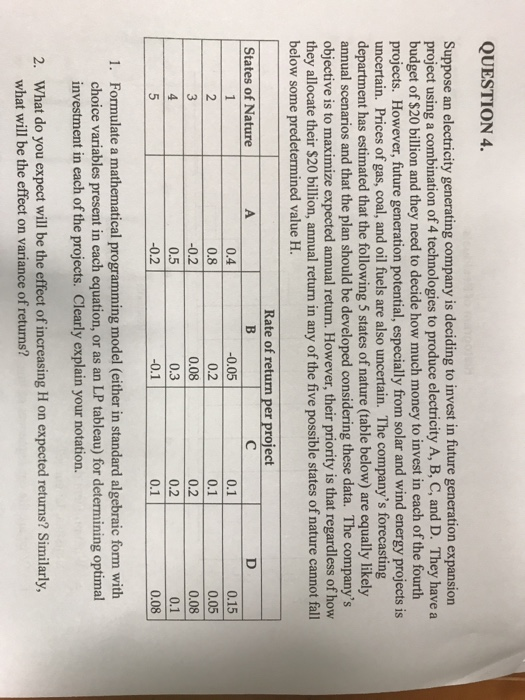# Answered! Suppose an electricity generating company is deciding to invest in future generation expansion project using a…Suppose an electricity generating company is deciding to invest in future generation expansion project using a combination of 4 technologies to produce electricity A, B, C, and D. They have a budget of \$20 billion and they need to decide how much money to invest in each of the fourth projects. However, future generation potential, especially from solar and wind energy projects is uncertain. Prices of gas, coal, and oil fuels are also uncertain. The company’s forecasting department has estimated that the following 5 states of nature (table below) are equally likely annual scenarios and that the plan should be developed considering these data. The company’s objective is to maximize expected annual return. However, their priority is that regardless of how they allocate their \$20 billion, annual return in any of the five possible states of nature cannot fall below some predetermined value H. Formulate a mathematical programming model (either in standard algebraic form with choice variables present in each equation, or as an LP tableau) for determining optimal investment in each of the projects. Clearly explain your notation. What do you expect will be the effect of increasing H on expected returns? Similarly, what will be the effect on variance of returns?

1.

LP Formulation

Let XA, XB, XC, and XD be the money invested in technology A, B, C, and D respectively.
Objective Function:
max.Z = Total Return
= 1/5{(0.4+0.8-0.2+0.5-0.2)*XA + (-0.05+0.2+0.08+0.3-0.1)*XB + (0.1+0.1+0.2+0.2+0.1)*XC + (0.15+0.05+0.08+0.1+0.08)*XD}
= 1/5{1.3*XA + 0.43*XB + 0.70*XC + 0.46*XD}
[Note that we don’t have to consider the 1/5 term as that is common to all]
Subject to,
XA + XB + XC + XD ≤ 20
0.4*XA – 0.05*XB + 0.1*XC + 0.15XD ≥ H
0.8*XA + 0.2*XB + 0.1*XC + 0.05XD ≥ H
-0.2*XA + 0.08*XB + 0.2*XC + 0.08XD ≥ H
0.50*XA + 0.3*XB + 0.2*XC + 0.10XD ≥ H
-0.2*XA – 0.1*XB + 0.1*XC + 0.08XD ≥ H
XA, XB, XC, XD ≥ 0

Don't use plagiarized sources. Get Your Custom Essay on
Answered! Suppose an electricity generating company is deciding to invest in future generation expansion project using a…
GET AN ESSAY WRITTEN FOR YOU FROM AS LOW AS \$13/PAGE

In a standard LP tableu, this will look like:

 Simplex Tableau CBi Cj 1.3 0.43 0.7 0.46 0 0 0 0 0 0 Solution Basic variables XA XB XC XD s1 s2 s3 s4 s5 s6 0 s1 -0.4 0.05 -0.1 -0.15 1 0 0 0 0 0 – H 0 s2 -0.8 -0.2 -0.1 -0.05 0 1 0 0 0 0 – H 0 s3 0.2 -0.08 -0.2 -0.08 0 0 1 0 0 0 – H 0 s4 -0.5 -0.3 -0.2 -0.1 0 0 0 1 0 0 – H 0 s5 0.2 0.1 -0.1 -0.08 0 0 0 0 1 0 – H 0 s6 1 1 1 1 1 0 0 0 0 1 20 Zj 0 0 0 0 0 0 0 0 0 0 0 Cj – Zj 1.3 0.43 0.7 0.46 0 0 0 0 0 0

2.

If we increase ‘H’, the return will reduce and then at a certain point there will be no feasible solution to the problem. In this problem, that particular value is 2. The overall variance will reduce as we increase the value of ‘H’ up to 2.0.